# 目标检测3 - Fast RCNN

## Fast R-CNN的改进

• 实现了end-to-end的单阶段训练，采用多任务损失函数将分类和回归任务作为一个整体进行训练：把最后一层的Softmax换成两个，一个是对区域的分类Softmax（包括背景），另一个是对bounding box的微调。
• RCNN提取特征给SVM训练时候需要中间要大量的磁盘空间存放特征，Fast R-CNN去掉了SVM这一步，所有的特征都暂存在显存中，就不需要额外的磁盘空间了。
• 并且，由于去掉了单独的SVM训练，所有层都可以fine-tune。
• 网络有两个输入，一个是整张图片，另一个是候选proposals算法产生的可能proposals的坐标。

• RoI pooling层### RoI pooling

• 将RoI区域的卷积特征拆分成HxW的网格（如VGG是7x7）
• 每个网格内的所有特征进行max pooling## Fast R-CNN训练流程

1. 采用预训练网络初始化

作者实验了三个pre-trained的ImageNet 网络：CaffeNet，VGG_CNN_M_1024，VGG-16，每个网络有5个max pooling层和5~13个卷积层。当用pre-trained的网络初始化Fast R-CNN网络时，要进行3步转化后再fine-tune：

1. 最后一个max pooling层由 RoI pooling层代替，该层将 H 和W 设置为与第一个全连接层兼容；
2. 网络最后那层全连接层和softmax（被训练用于包含1000类的ImageNet分类）被替换为前面描述的两个同级层：softmax的对K+1个类别的分类层，和bounding box 回归层
3. 网络有两个输入数据：一组原始图片和每张图片的一组RoIs
2. 特定样本下（自己的数据集）fine-tune for detection

Fast R-CNN可以用反向传播训练所有层的网络权重。作者提出了一种更有效的训练方法：利用训练期间的特征共享。在Fast RCNN训练中，随机梯度下降（SGD）小批量计算被分级采样，首先随机取样 $N$$N$ 张图片，然后每张图片取样 $R/N$$R / N$ 个RoIs 。

关键在于来自相同图像的RoI在向前和向后传播中共享计算和内存，这大大减少了一个mini-batch的计算。例如，当 $N=2,R=128$$N=2,R=128$时，这种方法比从128个不同的图像中提取一个RoI的方法要快64倍。

采用这种sample的方式是为了配合SPP的改进：同一张图片只用做一次卷积得到conv5 feature map，再把原图通过SS得到的proposal映射到feature map。而只有配合这种sample方式才可以发挥这个改进的优势。

这种策略的一个缺点在于其可能会减慢训练的收敛因为来自同一张图片的RoIs是相关的。但是在实践中这种担忧并没有出现，当 $N=2,R=128$$N=2,R=128$ 时，比RCNN使用更少的SGD迭代得到了更好的结果。

除了分层采样，Fast R-CNN使用一个精简的训练过程，一次fine-tune中联合优化softmax分类器和bbox回归器，而不是在三个独立的阶段训练softmax分类器，SVMs和Regressions。这一步包含了：

• 小批量取样（mini-batch sampling）
• RoI pooling层的反向传播（backpropagation through RoI pooling layers）
• SGD超参数（SGD hyperparameters）

### Fast R-CNN多任务损失函数

Fast R-CNN网络分类损失和回归损失如下图所示【仅针对一个RoI即一类物体说明】，黄色框表示训练数据，绿色框表示输入目标：• cls_score层用于分类，输出每个RoI输出在 $K+1$$K+1$ 个类别上的离散概率分布：$p=\left({p}_{0},\dots ,{p}_{K}\right)$$p=(p_0,…,p_K)$
• bbox_predict层用于调整候选区域位置，输出bounding box回归的offsets：${t}^{u}=\left({t}_{x}^{k},{t}_{y}^{k},{t}_{w}^{k},{t}_{h}^{k}\right)$$t^u=(t^k_x,t^k_y,t^k_w,t^k_h)$$k$$k$ 表示某个类别k，前两个参数是指相对于object proposal 尺度不变的平移 ，后两个参数是指log空间中相对于object proposal的高与宽。共$4\ast K$$4*K$维数组，也就是说对于每个类别都会训练一个单独的回归器

$u$$u$ 是每个用于训练的RoI标记的真实物体类别，$v$$v$ 是边界框回归目标的ground-truth，则：

• loss_cls层评估分类代价，由真实类别 $u$$u$ 对应的log loss决定：${L}_{cls}\left(p,u\right)=-log{p}_{u}$$L_{cls}(p,u)=-logp_u$

• loss_bbox评估回归损失代价，比较真实类别 $u$$u$ 对应的预测平移缩放参数 ${t}^{u}=\left({t}_{x}^{u},{t}_{y}^{u},{t}_{w}^{u},{t}_{h}^{u}\right)$$t^u=(t^u_x,t^u_y,t^u_w,t^u_h)$ 和真实平移缩放参数 $v=\left({v}_{x},{v}_{y},{v}_{w},{v}_{h}\right)$$v=(v_x,v_y,v_w,v_h)$ 的差距：

$L_{loc}(t^u,v)=\sum_{i\in\{x,y,w,h\}}smooth_{L_1}(t_i^u-v_i)\\smooth_{L_1}(x)=\begin{cases}0.5x^2, & \text{ |x|<1 } \\|x|-0.5, & \text{otherwise}\end{cases}$

$smoot{h}_{L1}$$smooth_{L1}$ 损失函数曲线如下图所示，相比于 $L2$$L2$ 损失函数，其对离群点、异常值不敏感，可控制梯度的量级使其训练时不容易跑飞（prevent exploding gradients）：$L(p,u,t^u,v)=L_{cls}(p,u)+\lambda[u\ge1]L_{loc}(t^u,v) \\ [u\ge1]=\begin{cases}1, & \text{u>1 } \\0, & \text{otherwise}\end{cases}$

• 约定 $u=0$$u=0$ 为背景类，那么 $\left[u\ge 1\right]$$[u≥1]$ 函数用于限制背景候选区域即负样本不参与回归损失，不需要对候选区域进行回归操作；
• $\lambda$$\lambda$ 控制分类损失和回归损失的平衡，文中所有实验 $\lambda =1$$λ=1$### Mini-batch sampling

• R-CNN和SPPnet中采用RoI-centric sampling：从所有图片的所有候选区域中均匀取样，这样每个SGD的mini-batch中包含了不同图像的样本，不同图像之间不能共享卷积计算和内存，运算开销大；
• Fast R-CNN中采用image-centric sampling： 每个mini-batch采用层次采样，即先对 $N$$N$ 张图像采样 ，再在采样到的图像中对候选区域采样（每个图像中采样 $R/N$$R/N$ 个，一个mini-batch共计 $R$$R$ 个候选区域样本），同一张图像的候选区域卷积共享计算和内存，降低了运算开销； image-centric sampling方式采样的候选区域来自于同一图像，相互之间存在相关性，可能会减慢训练收敛的速度，但是作者在实际实验中并没有出现这样的现象，反而使用 $N=2，R=128$$N=2，R=128$ 的RoI-centric sampling方式比R-CNN收敛更快。

### RoI Pooling层反向传播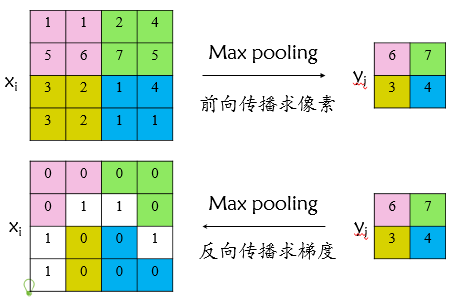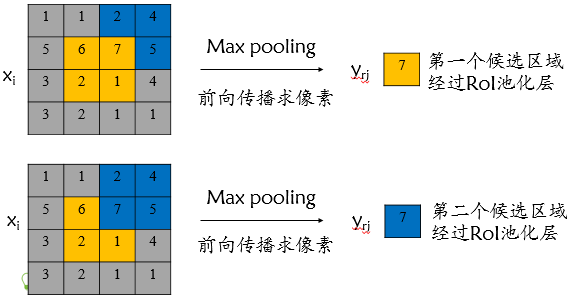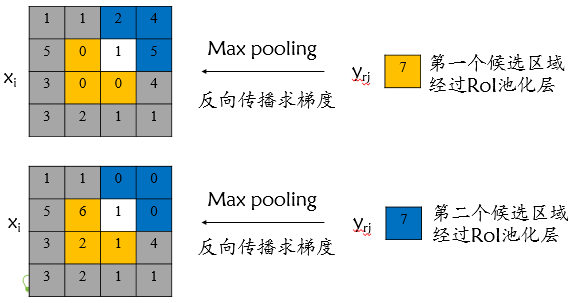$\frac{\mathrm{\partial }L}{\mathrm{\partial }{x}_{i}}=\sum _{r}\sum _{j}\left[i={i}^{\ast }\left(r,j\right)\right]\frac{\mathrm{\partial }L}{\mathrm{\partial }{y}_{rj}}$

### SGD超参数

• 用于分类的全连接层以均值为0、标准差为0.01的高斯分布初始化，用于回归的全连接层以均值为0、标准差为0.001的高斯分布初始化，偏置都初始化为0；
• 针对PASCAL VOC 2007和2012训练集，前30k次迭代全局 learning rate为0.001，每层权重学习率为1倍，偏置学习率为2倍，后10k次迭代全局学习率更新为0.0001；
• 动量momentum设置为0.9，权重衰减weight_decay设置为0.0005。

## Fast R-CNN测试流程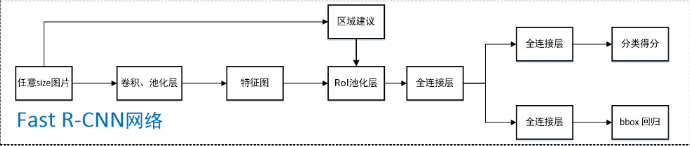1. 任意size图片输入CNN网络，经过若干卷积层与池化层，得到特征图；
2. 在任意size图片上采用selective search算法提取约2k个建议框；
3. 根据原图中建议框到特征图映射关系，在特征图中找到每个建议框对应的特征框（深度和特征图一致），并在RoI池化层中将每个特征框池化到H×W（VGG-16网络是7×7）的size；
4. 固定H×W大小的特征框经过全连接层得到固定大小的特征向量；
5. 第4步所得特征向量经由各自的全连接层（由SVD分解实现），分别得到两个输出向量：一个是softmax的分类得分，一个是Bounding-box窗口回归；
6. 利用窗口得分分别对每一类物体进行非极大值抑制剔除重叠建议框，最终得到每个类别中回归调整后得分最高的窗口。

【论文】一旦Fast R-CNN网络被微调，detection过程相当于运行正向传播（假设对象建议框object proposal是预先计算的）。网络将图像（或图像金字塔，编码为图像列表）和一组待给得分的 $R$$R$ 个对象建议框（object proposal）作为输入（R一般为2000）。当使用图像金字塔的时候，每个RoI被尺度归一化为接近 $224×224$$224\times224$ （意思可能是用RoI pooling操作模拟resize(224,224)？maybe 7x7在原图上的感受野是224x224）。对于每个测试RoI $r$$r$ ，网络输出关于 $r$$r$ 的一个后验概率分布 $p$$p$ 和一系列预测bbox偏移（每个类 [共K个类] 获得自己的精确bbox预测）。然后使用估计概率 $Pr\left(class=k|r\right)\triangleq {p}_{k}$$Pr(class=k|r)\triangleq p_k$$r$$r$ 赋予关于 $k$$k$ 个对象类的检测置信度。最后给每个类都实施一个非极大值抑制。

### Truncated SVD for faster detection

1. 物体分类和窗口回归都是通过全连接层实现的，假设全连接层输入数据为 $x$$x$ ，输出数据为 $y$$y$ ，全连接层参数为 $W$$W$ ，尺寸为 $u×v$$u×v$，那么该层全连接计算为 $y=Wx$$y = Wx$ ，计算复杂度为 $u×v$$u×v$

2. 若将 $W$$W$ 进行SVD分解，并用前 $t$$t$ 个特征值近似代替，即：

$W=U\sum {V}^{T}\approx U\left(u,1:t\right)\cdot \sum \left(1:t,1:t\right)\cdot V\left(v,1:t{\right)}^{T}$$W=U\sum V^T\approx U(u,1:t)\cdot \sum(1:t,1:t)\cdot V(v,1:t)^T$

那么原来的前向传播分解成两步：

$y=Wx=U\cdot \left(\sum \cdot {V}^{T}\right)\cdot x=U\cdot z$$y=Wx=U\cdot(\sum\cdot V^T)\cdot x=U\cdot z$

结合下图，先 $\left(\sum \cdot {V}^{T}\right)\cdot x$$(\sum\cdot V^T)\cdot x$ 得到 $z$$z$ ，再 $U\cdot z$$U\cdot z$ 得到 $y$$y$ ，计算复杂度为 $u×t+v×t$$u×t+v×t$（两步分解）,若 $t$t ，则这种分解会大大减少计算量；

在实现时，相当于把一个全连接层拆分为两个全连接层，第一个全连接层不含偏置，第二个全连接层含偏置；实验表明，SVD分解全连接层能使mAP只下降0.3%的情况下提升30%的速度，同时该方法也不必再执行额外的微调操作。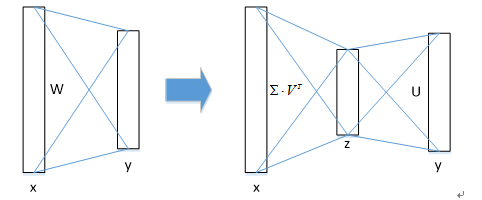### 思考

#### Q3：如何处理尺度不变性（scale invariance）？

• 单一尺度直接在训练和测试阶段将image预先固定好像素大小，直接输入网络训练就好，然后期望在训练过程中网络自己能够学习到尺度不变性scale-invariance；
• 多尺度在训练阶段随机从图像金字塔（缩放图片的scale得到，得到多尺度图片，相当于扩充数据集）中采样训练，通过一个图像金字塔向网络提供一个近似的尺度不变，在测试阶段图像金字塔用来对每个object proposal近似尺度归一化，训练阶段每次采样一个图像就随机采样一个金字塔尺度。

#### Q6：哪些layer的参数需要fine-tune？

SPPnet论文中采用ZFnet（AlexNet的改进版）这样的小网络，其在微调阶段仅对全连接层进行微调，就足以保证较高的精度，作者文中采用VGG-16网络，若仅仅只对全连接层进行微调，mAP会从66.9%降低到61.4%， 所以文中也需要对RoI池化层之前的卷积层进行微调；

## Fast R-CNN缺点

Fast R-CNN中采用selective search算法提取候选区域，而目标检测大多数时间都消耗在这里（selective search算法候选区域提取需要2~3s，而提特征分类只需要0.32s），这无法满足实时应用需求，而且Fast R-CNN并没有实现真正意义上的端到端训练模式（候选区域是使用selective search算法先提取出来的）；